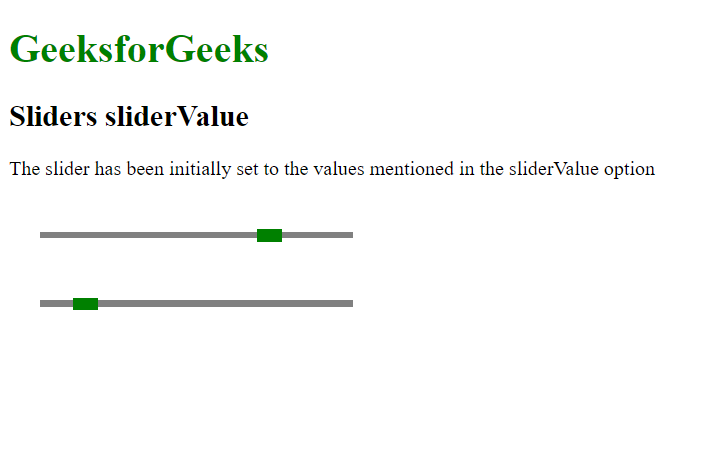# script.aculo.us Sliders sliderValue Option

• Last Updated : 20 Nov, 2020

The script.aculo.us library is a cross-browser library that aims to improving the user interface of a website. The Slider controls are thin tracks that allow the user to input values. It is done by defining a range of values that can be selected by the user by dragging the handle to the appropriate value.

The Sliders sliderValue option is used to define the initial value that the slider handle would be set. This is only followed when the given value is within the range of the values that the slider is allowed to represent.

Hey geek! The constant emerging technologies in the world of web development always keeps the excitement for this subject through the roof. But before you tackle the big projects, we suggest you start by learning the basics. Kickstart your web development journey by learning JS concepts with our JavaScript Course. Now at it's lowest price ever!

Syntax:

```{ sliderValue: value }
```

Values:

• value: This is a number that will be the initial value of the slider. The default value is 0.

Example

## HTML

 ```<``html``>`` ` `<``head``>``    ````    ``<``script` `type``=``"text/javascript"` `        ``src``=``"prototype.js"``>``    ```` ` `    ``<``script` `type``=``"text/javascript"` `        ``src``=``"scriptaculous.js?load = slider"``>``    ```` ` `    ````    ``<``style` `type``=``"text/css"``>``        ``.track {``            ``width: 250px;``            ``background-color: gray;``            ``height: 5px;``            ``position: relative;``        ``}`` ` `        ``.track .handle {``            ``width: 20px;``            ``height: 10px;``            ``background-color: green;``            ``cursor: move;``            ``position: absolute;``            ``top: -2px;``        ``}`` ` `        ``.pad {``            ``padding: 25px;``        ``}``    `````` ` `<``body``>``    ``<``h1` `style``=``"color: green;"``>``        ``GeeksforGeeks``    ```` ` `    ``<``h2``>Sliders sliderValue`` ` `    ``<``p``>``        ``The slider has been initially set``        ``to the values mentioned in the``        ``sliderValue option``    ```` ` `    ``<``div` `class``=``"pad"``>``        ``<``div` `id``=``"track-hor"` `class``=``"track"``>``            ``<``div` `id``=``"handle-hor"` `class``=``"handle"``>``            ````        ````    ```` ` `    ``<``div` `class``=``"pad"``>``        ``<``div` `id``=``"track2-hor"` `class``=``"track"``>``            ``<``div` `id``=``"handle2-hor"` `class``=``"handle"``>``            ````        ````    ```` ` `    ``<``script` `type``=``"text/javascript"``>``        ``new Control.Slider('handle-hor',``            ``'track-hor', {``            ``range: \$R(1, 10),`` ` `            ``// Set the initial value``            ``// of the slider``            ``sliderValue: 7.75,``        ``});`` ` `        ``new Control.Slider('handle2-hor',``            ``'track2-hor', {``            ``range: \$R(1, 10),`` ` `            ``// Set the initial value``            ``// of the slider``            ``sliderValue: 2,``        ``});``    `````` ` ``

Output:My Personal Notes arrow_drop_up